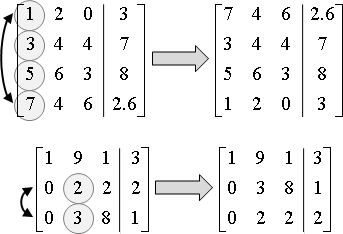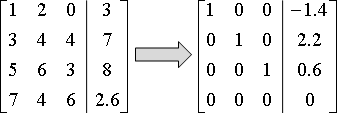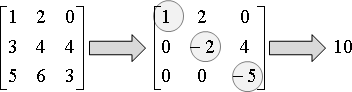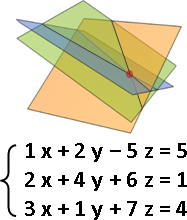Linear Equation

Linear Equation

「一次方程式」。一次函數的等式。

3 x + 4 y - 6 = 2 x + 5 z - 1

1 x + 2 y - 5 z = 5

System of Linear Equations

「一次方程組」。許多道一次方程式同時成立。

{ 1 x + 2 y - 5 z = 5
{ 2 x + 4 y + 6 z = 1
{ 3 x + 1 y + 7 z = 4

{ 1 x + 2 y - 5 z = 5        [ 1 2 -5 ] [ x ]   [ 5 ]
{ 2 x + 4 y + 6 z = 1  --->  [ 2 4  6 ] [ y ] = [ 1 ]
{ 3 x + 1 y + 7 z = 4        [ 3 1  7 ] [ z ]   [ 4 ]
A        x       b

Inverse

「反矩陣」。一旦知道反矩陣，即可輕鬆解一次方程組。而且還可以隨意抽換等號右邊數值。

solve Ax = b  --->  find A⁻¹, then x = A⁻¹b

Linear Equation: Eliminationpivot row：首項係數不是零的橫條。
pivot：上述橫條的首項係數。
pivoting：找到pivot row，視情況進行交換。UVa 10109 10524 10828 ICPC 3563

「高斯喬登消去法」是延伸版本。對角線化成一、其餘化成零。UVa 684

LU Decomposition

http://ccjou.wordpress.com/2010/09/01/

LU分解的用途是解大量一次方程組Ax = b，A固定，b有許多組。這種情況下，LU分解，每次求解需時O(N²)；高斯消去法，每次求解需時O(N³)。

Cholesky Decomposition

Linear Equation: Relaxation

[ 4  3 ] [ x ] = [ 1 ]
[ 2  5 ] [ y ]   [ 2 ]

{ 4x + 3y = 1  => { x = (1 - 3y) / 4
{ 2x + 5y = 2     { y = (2 - 2x) / 5

[ x₀ ] = [ 0 ] 隨便設定一解，作為初始值
[ y₀ ]   [ 0 ]

[ x₁ ] = [ (1 - 3y₀) / 4 ]
[ y₁ ]   [ (2 - 2x₀) / 5 ]

[ x₂ ] = [ (1 - 3y₁) / 4 ]
[ y₂ ]   [ (2 - 2x₁) / 5 ]

[ 4  3 -1 ] [ x ]   [ 1 ]
[ 2  5  1 ] [ y ] = [ 2 ]
[-2 -2  6 ] [ z ]   [ 3 ]

{  4x + 3y -  z = 1     { x = (1 - 3y +  z) / 4
{  2x + 5y +  z = 2  => { y = (2 - 2x -  z) / 5
{ -2x - 2y + 6z = 3     { z = (3 + 2x + 2y) / 6

[ x₀ ]   [ 0 ]
[ y₀ ] = [ 0 ] 隨便設定一解，作為初始值
[ z₀ ]   [ 0 ]

[ x₁ ]   [ (1 - 3y₀ +  z₀) / 4 ]
[ y₁ ] = [ (2 - 2x₀ -  z₀) / 5 ]
[ z₁ ]   [ (3 + 2x₀ + 2y₀) / 6 ]

Ax = b
(D+L+U)x = b                  D是對角線、L是嚴格下三角、U是嚴格上三角
Dx = b - (L+U)x
x = D⁻¹ [b - (L+U)x]
x = D⁻¹b - D⁻¹(L+U)x

「嚴格對角優勢strictly diagonally dominant」：每個橫條，對角線元素大於其餘元素總和；每個直條亦然。

for each row, |Aii| > ∑ |Aij|
j≠i

[ 4  3 -1 ] [ x ]   [ 1 ]
[ 2  5  1 ] [ y ] = [ 2 ]
[-2 -2  6 ] [ z ]   [ 3 ]

{  4x + 3y -  z = 1     { x = (1 - 3y +  z) / 4
{  2x + 5y +  z = 2  => { y = (2 - 2x -  z) / 5
{ -2x - 2y + 6z = 3     { z = (3 + 2x + 2y) / 6

[ x₀ ]   [ 0 ]
[ y₀ ] = [ 0 ] 隨便設定一個初始值
[ z₀ ]   [ 0 ]

[ x₁ ]   [ (1 - 3y₀ +  z₀) / 4 ] 依序計算x₁、y₁、z₁，
[ y₁ ] = [ (2 - 2x₁ -  z₀) / 5 ] 剛出爐的數字，馬上拿來使用，
[ z₁ ]   [ (3 + 2x₁ + 2y₁) / 6 ] 加快收斂速度。
xₖ₊₁ = D⁻¹ (b - Uxₖ - Lxₖ₊₁)

[ x₁ ]   [ (1-w) ⋅ x₀ + w ⋅ (1 - 3y₀ +  z₀) / 4 ]
[ y₁ ] = [ (1-w) ⋅ y₀ + w ⋅ (2 - 2x₁ -  z₀) / 5 ]
[ z₁ ]   [ (1-w) ⋅ z₀ + w ⋅ (3 + 2x₁ + 2y₁) / 6 ]

Linear Equation: Projection

Linear Equation與GeometryContourPlot3D[1 x + 2 y - 5 z == 5, {x, -5, 5}, {y, -5, 5}, {z, -5, 5}, Boxed -> False, Axes -> False, Mesh -> None]f := 1 x + 2 y - 5 z - 5; g := 2 x + 4 y + 6 z - 1; h := 3 x + 1 y + 7 z - 4; ContourPlot3D[{f == 0, g == 0, h == 0}, {x, -5, 5}, {y, -5, 5}, {z, -5, 5}, Boxed -> False, Axes -> False, Mesh -> None, ContourStyle -> Directive[Opacity[0.5]]]「克拉瑪公式」則是此演算法的高維度版本，形成了非常漂亮的公式解！求得超平行體的容積，以容積比例求得解。

linear equation:
Ax = b

solution:
{ x = det(Aˣ) / det(A)
{ y = det(Aʸ) / det(A)
{ z = det(Aᶻ) / det(A)

unisolvence:
Ax = b has a unique solution   iff   det(A) ≠ 0

example:
{ 1 x + 2 y - 5 z = 5        [ 1 2 -5 ] [ x ]   [ 5 ]
{ 2 x + 4 y + 6 z = 1  --->  [ 2 4  6 ] [ y ] = [ 1 ]
{ 3 x + 1 y + 7 z = 4        [ 3 1  7 ] [ z ]   [ 4 ]
A        x       b
_ b                  _ b                  _ b
[|5| 2 -5 ]       [ 1 |5|-5 ]       [ 1  2 |5|]
Aˣ = [|1| 4  6 ]  Aʸ = [ 2 |1| 6 ]  Aᶻ = [ 2  4 |1|]
[|4| 1  7 ]       [ 3 |4| 7 ]       [ 3  1 |4|]
‾                    ‾                    ‾

determinant是矩陣當中所有向量所構成的超平行體的容積。時間複雜度等於N+1次determinant的時間複雜度，O(N⁴)。

Determinant

determinant起初用來判定一個一次方程組是否有解、解是多少，因而稱作「決定因子」。古人沒有意識到determinant是容積。

http://mathworld.wolfram.com/DeterminantExpansionbyMinors.html

N = 2 or 3的時候比較特別，可以直接累加所有「左上右下斜線」的乘積、累減「右上左下斜線」的乘積。中學數學課程有教。

Linear Equation: Decomposition

Linear Equation與Algebra

{ 1 x + 2 y - 5 z = 5        [ 1 2 -5 ] [ x ]   [ 5 ]
{ 2 x + 4 y + 6 z = 1  --->  [ 2 4  6 ] [ y ] = [ 1 ]
{ 3 x + 1 y + 7 z = 4        [ 3 1  7 ] [ z ]   [ 4 ]
A        x       b

-1
[ 1 2 -5 ] [ x ]   [ 5 ]        [ x ]   [ 1 2 -5 ] [ 5 ]
[ 2 4  6 ] [ y ] = [ 1 ]  --->  [ y ] = [ 2 4  6 ] [ 1 ]
[ 3 1  7 ] [ z ]   [ 4 ]        [ z ]   [ 3 1  7 ] [ 4 ]
A        x       b            x         A⁻¹      b

solve Ax = b  --->  find A⁻¹, then x = A⁻¹b

A   = EΛE⁻¹
A⁻¹ = EΛ⁻¹E⁻¹
x = A⁻¹b = EΛ⁻¹E⁻¹b

Linear Equation: Root Finding

Ax = b的解，即是f(x) = Ax - b的根。

Ax = b的解，即是g(x) = Ax - b + x的不動點。

Linear Equation: Optimization

「一次函數求解」等於「二次函數求極值」

A必須是對稱正定矩陣，使得二次函數有唯一最小值。

minimize f(x) = 1/2 xᵀAx - bᵀx
solve f′(x) = Ax - b = 0
solve Ax = b

f(x)的最小值位置，即是f′(x)的根，即是Ax = b的解。

f(x)的最佳化演算法是「共軛梯度法」。優於高斯消去法。

「矩陣求解」化作「對稱正定矩陣求解」

solve Ax = b       (if A has unique solution)
---> solve AᵀAx = Aᵀb   (AᵀA is symmetric positive definite)
---> solve A'x = b'     (unique solution)
solve Ax = b       (if A has no solution)
---> min ‖Ax - b‖²      (least squares)
---> solve AᵀAx = Aᵀb   (normal equation)
---> solve A'x = b'     (least squares solution)

Linear Least Squares

Linear Least Squares

no solution：無解。改求‖Ax - b‖²最小的解。
infinitely many solutions：多解。改求‖x‖²最小的解。
unique solution：唯一解。求Ax = b的解。

min ‖Ax - b‖²：方程組每一道等式，求得等號左右兩邊的差的平方；累計所有等式，總和越小越好。

min ‖x‖²：解的每一項的平方，總和越小越好。

Least意指「盡量小」，Squares意指「平方和」。

No Solution / Infinitely Many Solutions

rank：消去冗餘的等式。column：直條數量、變數數量。

no solution              : rank(A) ≠ rank([A|b])
infinitely many solutions: rank(A) = rank([A|b]) ≠ column(A)
unique solution          : rank(A) = rank([A|b]) = column(A)

Overdetermined System / Underdetermined System

[ X X ]               [ X X X ]
[ X X ]   [ X X X ]   [ X X X ]
[ X X ]   [ X X X ]   [ X X X ]
thin        fat        square
overdetermined system  (thin matrix)  : row(A) > column(A)
underdetermined system (fat matrix)   : row(A) < column(A)
welldetermined system  (square matrix): row(A) = column(A)

Pseudoinverse（Moore–Penrose Inverse）

「虛擬反矩陣」、「偽反矩陣」。反矩陣從方陣推廣成矩陣。

solve Ax = b  --->  find A⁺, then x = A⁺b

Linear Least Squares: Decomposition

solve overdetermined system Ax = b  --->  min ‖Ax - b‖²

x = (Aᵀ A)⁻¹ Aᵀ b     ( Aᵀ A x = Aᵀ b )   Normal Equation

x = R⁻¹ Q b           ( A = Q R )         QR Decomposition

x = V Σ⁻¹ Uᵀ b        ( A = U Σ Vᵀ )      Singular Value Decomposition
solve underdetermined system Ax = b  --->  min ‖x‖²

x = Aᵀ (A Aᵀ)⁻¹ b                         Normal Equation

x = Q (Rᵀ)⁻¹ b        ( Aᵀ = Q R )        QR Decomposition

x = U Σ⁻¹ Vᵀ b        ( Aᵀ = U Σ Vᵀ )     Singular Value Decomposition

http://people.csail.mit.edu/bkph/articles/Pseudo_Inverse.pdf

「一次微分等於零」的地方是極值、鞍點。因為平方誤差是開口向上的拋物面，所以「一次微分等於零」的地方必是最小值。

solve Ax = b
min ‖Ax - b‖²
d/dx ‖Ax - b‖² = 0                    「一次微分等於零」的地方是極值、鞍點
[ d/dx (Ax - b) ] [ 2(Ax - b) ] = 0   微分連鎖律
Aᵀ [ 2(Ax - b) ] = 0                  微分
AᵀAx = Aᵀb                            同除以2、展開、移項
x = (AᵀA)⁻¹Aᵀb                        移項。注意到A的向量們必須線性獨立！

Aᵀb   dot(A,b)
x = (AᵀA)⁻¹Aᵀb = ――― = ―――――――― = projAb
AᵀA   dot(A,A)

solve Ax = b
---> min ‖Ax - b‖²
---> 2Aᵀ(Ax - b) = 0
---> x = projAb

http://www.cs.cornell.edu/courses/cs322/2007sp/notes/qr.pdf

solve Ax = b
min ‖Ax - b‖²
min ‖Qᵀ(Ax - b)‖²           正規正交矩陣，變換後長度不變
min ‖QᵀAx - Qᵀb‖²           展開
min ‖Rx - Qᵀb‖²             QᵀA = QᵀQR = R
min ‖ [ R₁x - Q₁ᵀb ] ‖²     R = [ R₁ ]  區分出零，讓R₁是方陣
‖ [   0 - Q₂ᵀb ] ‖          [ 0  ]  區分上段和下段
solve R₁x - Q₁ᵀb = 0        此式有唯一解，可為零（因此最小值是 ‖Q₂ᵀb‖²）
R₁x = Q₁ᵀb                  移項
x = R₁⁻¹Q₁ᵀb                移項

solve Ax = b
min ‖Ax - b‖²
min ‖Uᵀ(Ax - b)‖²        正規正交矩陣，變換後長度不變
min ‖UᵀAx - Uᵀb‖²        展開
min ‖ΣVᵀx - Uᵀb‖²        UᵀA = UᵀUΣVᵀ = ΣVᵀ
solve ΣVᵀx - Uᵀb = 0     此式有唯一解，可為零
ΣVᵀx = Uᵀb               移項
x = VΣ⁻¹Uᵀb              移項

Linear Least Squares: Optimization

Tikhonov Regularization

solve Ax = b   --->   min ‖Ax - b‖²   [overdetermined system]
solve Ax = b   --->   min ‖x‖²        [underdetermined system]

solve Ax = b   --->   min ‖Ax - b‖² + α f(x)   (α ≥ 0)
solve Ax = b   --->   min ‖x‖² + α f(x)   (α ≥ 0)

solve Ax = b   --->   min ‖Ax - b‖² + α ‖x‖²   (α ≥ 0)
d/dx [ ‖Ax - b‖² + α ‖x‖² ] = 0   「一次微分等於零」的地方是極值、鞍點
二次函數、恆正，必得最小值
2 AᵀA x - 2 Aᵀ b + 2 α x = 0      展開

( AᵀA + α I ) x = Aᵀ b            移項，左式即是 AᵀA 的對角線加上 α

Homogeneous Linear Equation

solve Ax = 0   --->   min ‖Ax‖² subject to ‖x‖² = 1
min ‖Ax‖² - λ ( ‖x‖² - 1 )             Lagrange multiplier

∂/∂x [ ‖Ax‖² - λ ( ‖x‖² - 1 ) ] = 0   「一次微分等於零」的地方是極值、鞍點
二次函數，必得極值
2 AᵀA x - 2 λ x = 0                    展開

AᵀA x = λ x                            移項，此即特徵向量的格式

AᵀA是對稱半正定矩陣，特徵值即是奇異值，特徵向量即是奇異向量。

AᵀA的特徵值即是A的奇異值，AᵀA的特徵向量即是A的右奇異向量。

Linear Inequality

System of Linear Inequalities

「一次不等式組」。許多道一次不等式同時成立。